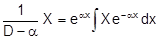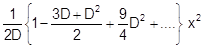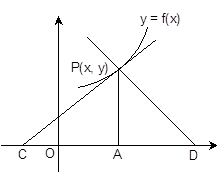×#### Thank you for registering.

One of our academic counsellors will contact you within 1 working day.

Click to Chat

1800-1023-196

+91-120-4616500

CART 0

• 0

MY CART (5)

Use Coupon: CART20 and get 20% off on all online Study Material

ITEM
DETAILS
MRP
DISCOUNT
FINAL PRICE
Total Price: Rs.

There are no items in this cart.
Continue Shopping```Methods of Finding Particular IntegralThere are different methods of finding the particular integral. The method to be applied depends on the type of function involved. There is a general method which can be applied to any kind of question but it is comparatively lengthy. The short methods are also there which are specific according to the type of function involved.

General Method

If both m1 and m2 are constants, the expressions (D – m1) (D – m2) y and (D– m2) (D – m1) y are equivalent i.e. the expression is independent of the order of operational factors.We will explain the method with the help of following

Example: Solve d2y/ dx2 – 5 dy/dx +6y = e3x.

Solution:

The equation can be written as

(D2 – 5D + 6)y = e3x

(D – 3) (D – 2)y = e3x

C.F.  = c1 e3x + c2e2x

And P.I. =1/ (D-3). 1/ (D-2) e3x

= 1/ (D-3) e2x ∫ e3x e-2xdx

=1/ (D-3) e2x ex

= e3x ∫ e3x e-3x dx = x.e3x

y = c1e3x +c2e2x +xe3x

P.I. can be found by resolving

1/f (D) = 1/ (D-3). 1/ (D-2)

Now using partial fractions,

1/f (D) = 1/ (D-3). 1/ (D-2)

= 1/ (D-3) – 1/ (D-2)

Hence, the required P.I. is [1(D-3) – 1/ (D-2)] e3x

= 1/ (D-3) e3x – 1/ (D-2) e3x

= e3x ∫ e3x e-3x dx – e2x ∫ e3x e-2x dx

= xe3x – e3x

Second term can be neglected as it is included in the first term of the C.F.

Short Method of Finding P.I.

In certain cases, the P.I.  can be obtained by methods shorter than the general method.

(i). To find P.I.  when  X = eax in f(D) y = X, where  a is constant

y = 1/f(D)

1/f(D) eax = 1/f(a) eax , if f(a) ≠ 0.

1/f(D) eax = xr/f r(a) eax , if f(a) = 0, where f(D) = (D-a)rf(D)

Example:  Solve (D3 –  5D2 + 7D – 3)y = e3x.

Solution:

(D – 1)2 (D – 3) y = e3x

C.F.  = aex + bx ex + ce3x

And P.I.  = 1/ (D3-5D2+7D-3) e3x

= x/ (3D2-10D+7) e3x

= ¼ xe3x

y = aex + bx ex + ce3x  + ¼ xe3x.

(ii). To find P.I.  when X = cos ax or sin ax

f (D) y = X

y = 1/f(D) sinax

If f (– a2) ≠ 0   then 1/f(D2) sinax = 1/f(-a2) sinax

If f (– a2) = 0 then (D2 + a2) is at least one factor of f (D2)

Let f (D2) = (D2 + a2)r f (D2)

Where f (– a2) ≠ 0

[1/f (D2)] sin ax = 1/ (D2+a2). 1/φ(D2) sin ax

= 1/ φ(-a2). 1/ (D2+a2) sin ax

Now, when r=1, 1/(D2 + a2) sin ax = -x/2a. cos ax

Similarly If  f(–a)2 ≠ 0  then 1/f(D2) cos ax = 1/f(-a2) cosax

And 1/ (D2+a2) cos ax = x/2a .sin ax

Example: Solve (D2 – 5D + 6) y = sin3x.

Solution: We can factorize the given term as (D – 2) (D – 3)y = sin3x

C.F.  = ae2x + be3x

P.I. = 1/ (D2 -5D +6) sin 3x

= 1/ [(-9) -5D +6] sin 3x

= 1/ (-5D-3) sin 3x

= - (5D-3). 1/ (25D2 -9) sin3x

= 1/234 (15 cos 3x-3 sin3x)

= ae2x + be3x + 1/234 (15 cos 3x-3 sin3x)

(iii) To find the P.I.  when X = xm  where m ∈ N

f (D) y = xm

y = 1/ f(D) xm

We will explain the method by taking an example

Example: Find P.I. of (D3 + 3D2 + 2D) y = x2.

Solution: The P.I. is given by 1/ (D3+3D2+2D) x2

==== 1/2D (1-3D/2+7/4D2 + ….) x2

= 1/2D (x2-3x +7/2)

And 1/2D (x2-3x+7/2) = 1/2(x3/3-3x2/2+7/2x) = 1/12 (2x3 -9x2+21x).

(iv) To find the value of 1/f(D) eax V where ‘a’ is a constant and V is a function of x

1/f (D) .eax V = eax.1/f (D+a). V

Example: Solve (D2 + 2) y = x2 e3x.

Solution: C.F.  = a cos √2x + b sin √2x

P.I. = 1/ (D2+2). x2e3x = e3x. 1/ [(D+3)2 +2] .x2

= e3x. 1/(D2 +6D+11). x2

= 1/11. e3x (1+ (6D+D2)/11)-1 x2

= 1/11 e3x [1- (6D+D2)/11 + 36/121 . D2 +…) x2

= 1/11 e3x (1-6D/11+25/121 . D2+…) x2

= 1/11 e3x (x2 – 12/11 x + 50/121)

= a cos √2x + b sin √2x + e3x/11 (x2-12/11 x +50/121)

(v). To find 1/f (D). xV where  V is a function of x

1/f (D). xV = [x- 1/f(D). f'(D)] 1/f(D) V

Watch this Video for more reference

Example: Solve (D2 + 4) y = x sin2x.

Solution: C.F.  = c1 cos 2x + c2 sin2x

P.I. = 1/ (D2+4). x sin 2x

= {x- 1/ (D2+4) .2D}. 1/ (D2+4). sin 2x

= {x- 1/ (D2+4). 2D} {-x/4 cos 2x}

= -x2/4 cos 2x + ½.1/(D2+4) (cos 2x- 2x sin 2x)

= -x2/4 .cos 2x + ½ 1/(D2+4) cos 2x – 1/(D2+4) x sin 2x

= -x2/8 cos 2x +1/16 x sin 2x

So, y= c1 cos 2x + c2 sin 2x – x2/ 8 cos 2x + 1/16 x sin 2x.

Some Results on Tangents and Normals:

(i) The equation of the tangent at P(x, y)   to the curve y= f(x)

is Y – y =  dy/dx(X-x)

(ii) The equation  of the  normal  at point P(x, y)  to the  curve y = f(x) is

Y – y =  [-1/ (dy/dx) ].(X – x )

(iii) The length of the tangent  = CP  =

y √[1+(dx/dy)2]

(iv) The  length of the normal = PD =

y √[1+(dy/dx)2](v)  The length of the Cartesian  subtangent  = CA = y dy/dx

(vi) The length of the Cartesian subnormal = AD = y dy/dx

(viii) The initial ordinate of the tangent = OB = y – x.dy/dx

The methods of finding P.I. are quite simple and with a bit of practice they can easily fetch you 2-3 questions in the JEE. You may also consult the Sample Papers to get an idea about the types of questions asked.

To read more, Buy study materials of Differential Equations comprising study notes, revision notes, video lectures, previous year solved questions etc. Also browse for more study materials on Mathematics here.
```### Course Features

• 731 Video Lectures
• Revision Notes
• Previous Year Papers
• Mind Map
• Study Planner
• NCERT Solutions
• Discussion Forum
• Test paper with Video Solution